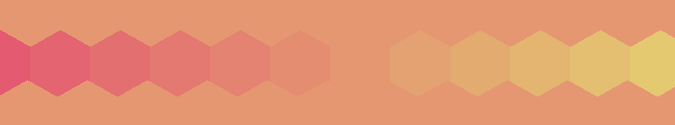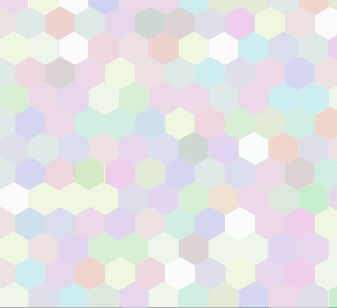# Perception of colour and contrast.I was looking up a 'Computer Recreations' article in September 1986 Scientific American, when I noticed this article on developments in theories oh how the brain interprets what the eye detects'. Main conclusion, then novel, was that the neighbourhood, and the amount of 'difference' within it, make recognising a pattern as significant either easier or harder.

It looked fun to apply my existing code, and I first produced sheets of various repeated colours. I then started colour-filling them with shades of colour.

One immediately obvious effect is that the brain sees step changes in chromaticity/brightness in an unexpected way. A step change between grey or colored regions is PERCEIVED as modulated near the boundary. An area of uniform chromaticity looks increasingly bright as you near a boundary with a darker area, and as you cross into the darker area it at first looks ub=nexpectedly brighter. An effect known to classicaal artists for centuries. This can give some of my images the appearance of being embossed, as the effect can mimic the brightness/shadow of a textured surface with side illumination. This effect particularly visible in the top sample shown below- although the middle strip is identical each time, making upper and lower sections uniform reduces but does not remove the effect.--The gifs below cycle through just some of my experimental variations.-## LB code for one variation.

This re-uses my code that replaces the inaccurate in-built turtle graphic commands of LB and adds a fill command. Shapes are represented in a string of 'how far to go forward' and 'now turn through angle' instructions. They assume you start near the centre of a closed figure, and can be scaled/rotated. The first datum holds the number of points to visit- eg for a triangle you have the centre, visit in turn three vertices. For a square you start at the centre, then move to the mid point of the upper edge, then move to four corners and on again to upper mid-point. The last turn is not needed.

```
'   **************************************************************************
'   ***                                                                     **
'   ***      colourIllusions8.bas    5 Jan 2020    tenochtitlanuk           **
'   ***                                                                     **
'   **************************************************************************

'   Demonstrates turtle drawn directly, avoiding LB's turtle errors and giving

'   Also demonstrates colour-filling. Shape outlines are drawn in a colour
'       differing by +1 in RGB components. 1 in 2^24 chance it's already in use!

'   The first argument for ExtFloodFill is the handle for the graphicbox device contest.

'   The x and y location to begin the fill are the next arguments.
'       These coordinates are counted from the upper left corner of the graphics area.

'   The arguments for color and fill type are next.

'   With _FLOODFILLBORDER ( =0) type of fill, the fill area is bounded by the color specified by the crColor parameter.

'   With _FLOODFILLSURFACE ( =1) type of fill, the fill area is defined by the color that is specified by crColor.
'       Filling continues outward in all directions as long as the color is encountered.
'       NB Fills from a centre within shape, which must be therefore on-screen.

nomainwin

global pi, TX, TY, Ttheta, wFillType, hdc

TX =400: TY =350: Ttheta =0 '   screen centre, pointing North/up.

pi =4 *atn( 1)

'        n      step  turn ..........

'shape\$ ="6,     0.5,  90,     0.5,  90,      1, 90,       1,  90,     1,   90,     0.5,  90"    ' square h/v oriented
shape\$ ="8,        1, 120,  1, 60,  1, 60,  1, 60,  1, 60,  1, 60,  1, 60,  1, 120"    '  hexagons
'add an 'R' flag if figure is a loop of identical step/turns??

WindowWidth  =750: WindowHeight =740    '   width 1150 if enable the textwindow for diagnostics

graphicbox #w.gb,  10, 10, 723, 704
'texteditor #w.te, 820, 10, 320, 700

open "Demo. of Filled Shapes & improved Turtle." for window as #w

#w    "trapclose quit"
#w.gb "down ; fill 80 80 80 ; color cyan"
'#w.te "!font courier 16"

h           = hwnd( #w.gb)
calldll #user32, "GetDC", h as ulong, hdc as ulong

wFillType   =0

'call graticule

#w.gb "flush ; up ; size 1"

oddLine =0

for y =0 to 89 step 5
Y   =int( y *10)

for x =0 to 79 step 5
scan
R           =int( 90 +y *2.5)
G           =int( 90 +x *2)
B           =int( 250 -y *2.5)'  +40 *rnd( 1))'   RGB never =255...
crColor     =MakeRGB( R, G, B)
#w.gb "color "; R; " "; G; " "; B    ' targetcolour R G B

if oddLine <>0 then X =int( 12 *x) else X =int( 12 *x +27)

'sub display shape\$, scale,  col\$,                                   x, y,  angle
call display shape\$, 34,     str\$( R) +" " +str\$( G) +" " +str\$( B), X, Y,  0  '   here, a single call.

#w.gb "backcolor "; R +1; " "; G +1; " "; B +1  '   visibly same, but distinguished by ExtFloodFill as boundary. -fillcolour   R+1 G+1 B+1

calldll #gdi32, "ExtFloodFill",_
hdc         As uLong,_      'device context
X           As Long,_       'x location to start filling
Y           As Long,_       'y location to start filling
crColor     As Long,_       'long colour value of border, or colour to replace
wFillType   As Long,_       'flag for type of fill
result      As Long         'nonzero if successful

'call display shape\$, 33, "200 170 200", X, Y, 0    '   to add outline if wanted
'call delay 1000
next x

if oddLine =0 then oddLine =1 else oddLine =0
next y

wait

'#w.te " Now at "+ using( "####", TX) +using( "####", TY) +" & facing " + using( "####.#", Ttheta)

#w.gb "flush"

wait

function MakeRGB( red, green, blue)
if red   <  0 then red   =  0
if red   >255 then red   =255
if green <  0 then green =  0
if green >255 then green =255
if blue  <  0 then blue  =  0
if blue  >255 then blue  =255

MakeRGB =( blue *256 *256) +( green *256) +red
end function

end function

end function

sub draw lifted, x, y
if lifted =0 then #w.gb "up" else #w.gb "down"
#w.gb "line "; TX; " "; TY; " "; x; " "; y
Ttheta  =atan2( x -TX, TY -y) *180 /pi  '   NB DEGREES.
TX      =x
TY      =y
end sub

sub turn angle  '   increment/update global turtle direction ( in DEGREES)
Ttheta =( Ttheta +angle) mod 360
end sub

sub forward s
#w.gb "down ; line "; TX; " "; TY; " "; TX +dx; " "; TY +dy; " ; up"
TX =TX +dx
TY =TY +dy
end sub

function atan2( x, y)
Result\$ = "Undetermined"
If ( x = 0) and ( y > 0) Then atan2 = pi / 2:     Result\$ = "Determined"
If ( x = 0) and ( y < 0) Then atan2 = 3 * pi / 2: Result\$ = "Determined"
If ( x > 0) and ( y = 0) Then atan2 = 0:          Result\$ = "Determined"
If ( x < 0) and ( y = 0) Then atan2 = pi:         Result\$ = "Determined"

If Result\$ = "Determined" Then [End.of.function]

BaseAngle = Atn( abs( y) /abs( x))

If (x > 0) and (y > 0) Then atan2 =        BaseAngle
If (x < 0) and (y > 0) Then atan2 = pi    -BaseAngle
If (x < 0) and (y < 0) Then atan2 = pi    +BaseAngle
If (x > 0) and (y < 0) Then atan2 = 2*pi  -BaseAngle
[End.of.function]
end function

sub graticule
for x =0 to 800 step 100    '   draw vertical graticule lines
#w.gb "line "; x; " "; 0; " "; x;   " "; 700
next x

for y =0 to 700 step 100
#w.gb "line "; 0; " "; y; " "; 800; " "; y
next y
end sub

[save]
#w.gb "flush ; getbmp scr 0 0 680 680"
filedialog "Save as ", "*.bmp", fn\$
bmpsave "scr", fn\$
wait

sub quit h\$
close #h\$
calldll #user32, "ReleaseDC", hw as ulong, hdc as ulong   'release the DC
end
end sub

'   _________________________________________

sub ellipse x, y, Major, Minor, inclination, R, G, B
crColor     =MakeRGB( R, G, B)

#w.gb "goto "; xs; " "; ys
#w.gb "down"
#w.gb "color "; R; " "; G; " "; B    ' targetcolour R G B
#w.gb "backcolor "; R +1; " "; G +1; " "; B +1  '   visibly same, but distinguished by ExtFloodFill as boundary. -fillcolour   R+1 G+1 B+1

for angle =0 to 370 step 10

#w.gb "goto "; xs; " "; ys
next angle

#w.gb "color red ; size 5 ; set "; x; " "; y; " ; size 1"
'call delay 1000
'goto [noFill]

calldll #gdi32, "ExtFloodFill",_
hdc         As uLong,_      'device context
x           As Long,_       'x location to start filling
y           As Long,_       'y location to start filling
crColor     As Long,_       'long colour value of border, or colour to replace
wFillType   As Long,_       'flag for type of fill
result      As Long         'nonzero if successful

[noFill]

#w.gb "up"

end sub

'n      d   tu    d   tu    d  tu ..
sub display shape\$, scale, col\$, x, y, angle    '   shape\$ ="5,    0.5,150,   1, 120,   1,120,   1,120,    1, 150"

noOfTerms =val( word\$( shape\$, 1, ","))

#w.gb "color "; col\$
#w.gb "north"

#w.gb "up ; goto "; x ; " "; y
#w.gb "turn "; angle

vStep  =val( word\$( shape\$, 2, ",")) *scale

#w.gb "go "; vStep:            print "up ; go "; vStep

vAngle =val( word\$( shape\$, 3, ","))

#w.gb "turn "; vAngle:         print "turn "; vAngle
#w.gb "down":                  print "down"

for i =1 to noOfTerms +1
vStep  =val( word\$( shape\$, 2 *i +2, ",")) *scale
vAngle =val( word\$( shape\$, 2 *i +3, ","))

#w.gb "go ";    vStep
#w.gb "turn ";  vAngle

print "go "; vStep; " ; turn "; vAngle
next i

'call delay 1000

end sub

sub delay t
timer t, [cont]
wait
[cont]
timer 0
end sub
```